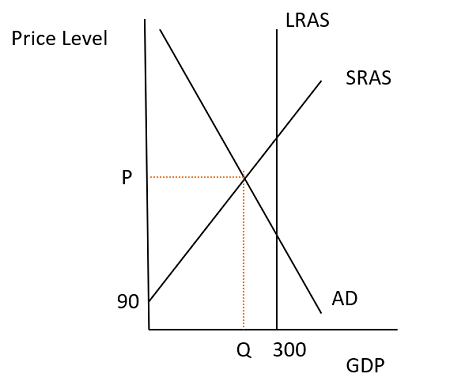# 0 100 200 300 400 500 90 100 110 120 130 140 150 Real GDP (billions of 2009 dollars)...

## Question:

0

100

200

300

400

500

90

100

110

120

130

140

150

Real GDP (billions of 2009 dollars)

Price level (GDP deflator)

aAD AD AS AS

The graph shows the aggregate demand curve, AD, and the? short-run aggregate supply? curve, AS?, in the economy of Artica. Potential GDP is \$300 billion.

Draw the potential GDP line. Label it.

Draw a point at the equilibrium price level and real GDP.

Initially, Artica has _.

A. an unemployment problem

B. zero unemployment because aggregate supply equals aggregate demand

C. an inflation problem

D. a federal funds rate that is set too low

## Aggregate Demand and Aggregate Supply:

Aggregate demand is the relationship between the price level and the quantity of real GDP demanded while aggregate supply is the relationship between the price level and the quantity of real GDP supplied.

## Answer and Explanation:

GDP and the price level in the short run are determined at the point where aggregate demand (AD) and the short-run aggregate supply (SRAS). In the long run, however, GDP is guided to potential GDP, which is the level of GDP that is achieved when the economy is operating at full employment. If the current equilibrium point is below potential GDP, which is also the long-run aggregate supply (LRAS), there is a recessionary GDP and there is unemployment. If, on the other hand, AD and SRAS intercept above potential GDP, there is an inflationary GAP and inflation is high.

It is not clear in this question what numbers coincide with which category (price level, aggregate supply, aggregate demand), so I drew a situation where the equilibrium is currently below potential GDP. In this situation, the correct answer is (A) an unemployment problem because there are fewer workers employed than would be employed if the economy was operating at full employment.#### Learn more about this topic:Aggregate Supply and Aggregate Demand (AS-AD) Model

from Economics 102: Macroeconomics

Chapter 7 / Lesson 3
58K